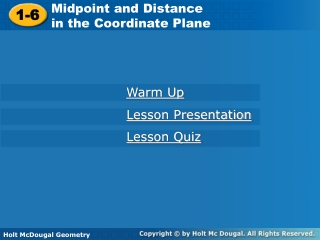DownloadDownload Presentation1-6

# 1-6

Télécharger la présentation## 1-6

- - - - - - - - - - - - - - - - - - - - - - - - - - - E N D - - - - - - - - - - - - - - - - - - - - - - - - - - -
##### Presentation Transcript

1. Midpoint and Distance in the Coordinate Plane 1-6 Warm Up Lesson Presentation Lesson Quiz Holt McDougal Geometry Holt Geometry

2. Warm Up • 1. Graph A (–2, 3) and B (1, 0). 2. Find CD. 8 3. Find the coordinate of the midpoint of CD. –2 4. Simplify. 4

3. Objectives Develop and apply the formula for midpoint. Use the Distance Formula and the Pythagorean Theorem to find the distance between two points.

4. Vocabulary coordinate plane leg hypotenuse

5. A coordinate planeis a plane that is divided into four regions by a horizontal line (x-axis) and a vertical line (y-axis) . The location, or coordinates, of a point are given by an ordered pair (x, y).

6. You can find the midpoint of a segment by using the coordinates of its endpoints. Calculate the average of the x-coordinates and the average of the y-coordinates of the endpoints.

7. Example 1: Finding the Coordinates of a Midpoint Find the coordinates of the midpoint of PQ with endpoints P(–8, 3) and Q(–2, 7). = (–5, 5)

8. Step 2 Use the Midpoint Formula: Example 2: Finding the Coordinates of an Endpoint M is the midpoint of XY. X has coordinates (2, 7) and M has coordinates (6, 1). Find the coordinates of Y. Step 1 Let the coordinates of Y equal (x, y).

9. – 2 – 7 –2 –7 Example 2 Continued Step 3 Find the x-coordinate. Set the coordinates equal. Multiply both sides by 2. 12 = 2 + x Simplify. 2 = 7 + y Subtract. –5 = y 10 = x Simplify. The coordinates of Y are (10, –5).

10. The Ruler Postulate can be used to find the distance between two points on a number line. The Distance Formula is used to calculate the distance between two points in a coordinate plane.

11. Find FG and JK. Then determine whether FG  JK. Example 3: Using the Distance Formula Step 1 Find the coordinates of each point. F(1, 2), G(5, 5), J(–4, 0), K(–1, –3)

12. Example 3 Continued Step 2 Use the Distance Formula.